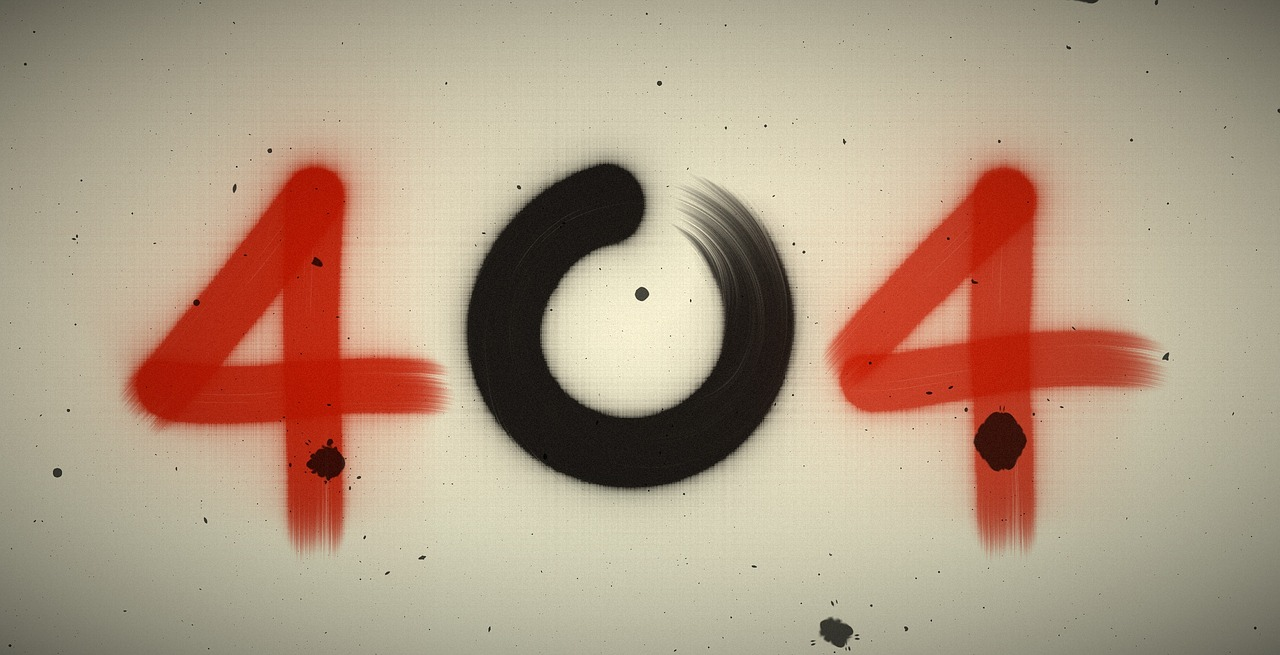## Post per Page

As being a physicist people ask about relationship between mathematics and physics. People ask why they are linked. Physics beautifully explains laws and rules of universe but when a physicist try it to explain in detail with equations most of people get bored and lose their interest in particular topic. So question here is what is the relationship between physics and mathematics? If you look briefly into the history of physics and mathematics and evolution of science you may find that sometimes physics leads, sometimes mathematics and sometimes they come together. Let me take you back in 1600s when Isaac newton asked a question that if the apple falls than does the moon also fall? This question at that time, was the greatest question ever asked by man. Answer by newton was “yes it does”. Gravity of earth pulls an apple and moon towards its center. So when he tried to explain the falling moon than he didn’t find any mathematics to solve this problem. Isaac newton then invented Calculus in order to explain the falling moon. Even now when you study calculus you first study the motion of falling body with the help of calculus and which is exactly how newton calculated motion of falling moon. Mathematics helped to calculate the motion of celestial bodies, so in a way we can say that mathematics and physics acted like twins in order to explain a practical question.

Image Credit- Donald E. Simanek

Einstein’s view of gravity was amazing and he explained it in a different way. Einstein’s question was “what’s the nature of origin of gravity?” Einstein explained gravity as a byproduct of curved space. So you are right now sitting on a chair and reading this article “why sitting on this chair?” Your answer might be that gravity of earth is pulling you down towards chair. If your answer is the same as I mentioned then Einstein must doubt about your answer. Because the reality is, earth has curved the space around you and curved space all around you is pushing you down on the chair. Pushing of space and time requires differential calculus. Differential calculus is a language of curved surfaces. The theory of curved surfaces that is differential equations came first and Einstein using differential equations explained physics of curved space-time.

In 1905 when Einstein proposed special relativity, mathematicians were working on topologies of higher dimension.  It was about 100 years ago and mathematics and physics parted different ways. Mathematics went into hyper space and that area of mathematics had nothing to do with physics of that time.  Physics plotted along for decades and we worked on atomic bombs, stars and laser beams but recently physicists discovered string theory. String theory can live in tenth and even eleventh dimension of hyper space. String theory evolved new kind of numbers that mathematicians never talked about and that’s why scientists call it super string theory. So we can say that now suddenly a new form of mathematics has evolved out of physics.

Goal of physics is to find a small equation which allows us to unify all the forces in the universe. Using mathematics and physics together we someday may be able of doing that, but so far it looks impossible. Both physics and mathematics can help us to reach our goals. Physics is useless without mathematics and without physics you cannot explain mathematics. So if you want to study physics and you are afraid of complex mathematics then keep one thing in mind, both physics and mathematics are the only keys towards an ultimate understanding of the entire physical universe.

## Error Page Image# Oooops.... Could not find it!!!

The page you were looking for, could not be found. You may have typed the address incorrectly or you may have used an outdated link.